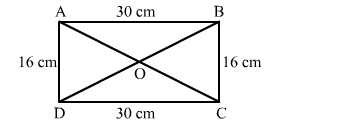# Find the length of a diagonal of a rectangle whose adjacent sides are 30 cm and 16 cm.

Question:

Find the length of a diagonal of a rectangle whose adjacent sides are 30 cm and 16 cm.

Solution:

Let ABCD be the rectangle with diagonals AC and BD meeting at O.
According to the question:
AB = CD = 30 cm and BC = AD = 16 cmApplying Pythagoras theorem in right-angled triangle ABC, we get:

$A C^{2}=A B^{2}+B C^{2}=30^{2}+16^{2}=900+256=1156$

$A C=\sqrt{1156}=34 \mathrm{~cm}$

Diagonals of a rectangle are equal.
Therefore, AC = BD = 34 cm